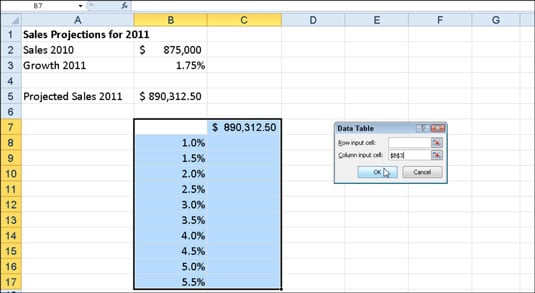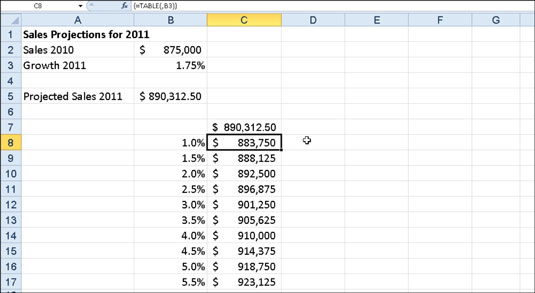##### Excel 2010 For DummiesTo create a one-variable data table to perform what-if analysis in Excel 2010, you enter a series of input values either across columns of a single row or down the rows of a single column. The formulas in a one-variable data table refer to only one input cell: a row input cell for input values entered across a row or a column input cell for input values entered down a column.

The steps below for creating a one-variable data table follow a specific example (rather than using generic steps) to help you understand exactly how to use this feature in your worksheets. The following figure shows a Sales Projections worksheet for which a one-variable data table is to be created. In this worksheet, the projected sales amount in cell B5 is calculated by adding last year's sales total in cell B2 to the amount that we expect it to grow in 2011 (calculated by multiplying last year's total in cell B2 by the growth percentage in cell B3).Sales projection worksheet with a column of possible growth percentages to be plugged into a one-variable data table.

The column of possible growth rates ranging from 1% all the way to 5.5% is entered down column B in the range B8:B17. Follow these steps to create a one-variable data table that plugs each of these values into the sales growth formula:

1. Copy the original formula entered in cell B5 into cell C7 by typing = (equal to) and then clicking cell B5.

The copy of the original formula (into which the series of different growth rates in B8:B17 is to be substituted) is now the column heading for the one-variable data table.

2. Select the cell range B7:C17.

The range of the data table includes the formula along with the various growth rates.

3. Choose What-If Analysis→Data Table in the Data Tools group on the Data tab.

Excel opens the Data Table dialog box.

4. Click in the Column Input Cell text box and then click cell B3, the Growth 2011 cell with the original percentage.

Excel inserts the absolute cell address, \$B\$3, into the Column Input Cell text box.

5. Click OK.

Excel creates the data table in the range C8:C17 by entering a formula using the TABLE function into this range. Each copy of this formula in the data table uses the growth rate percentage in the same row in column B to determine the possible outcome.Sales projection worksheet after creating the one-variable data table in the range C8:C17.

If you try to delete any single TABLE formula in the cell range C8:C17, Excel displays a Cannot Change Part of a Data Table alert dialog box — you must select the entire range of formulas (C8:C17 in this case) before you press Delete or click the Clear or Delete button on the Home tab.